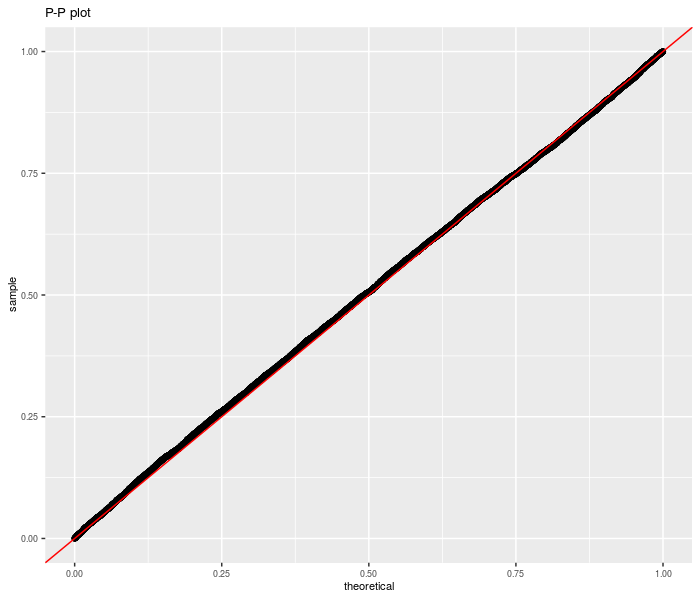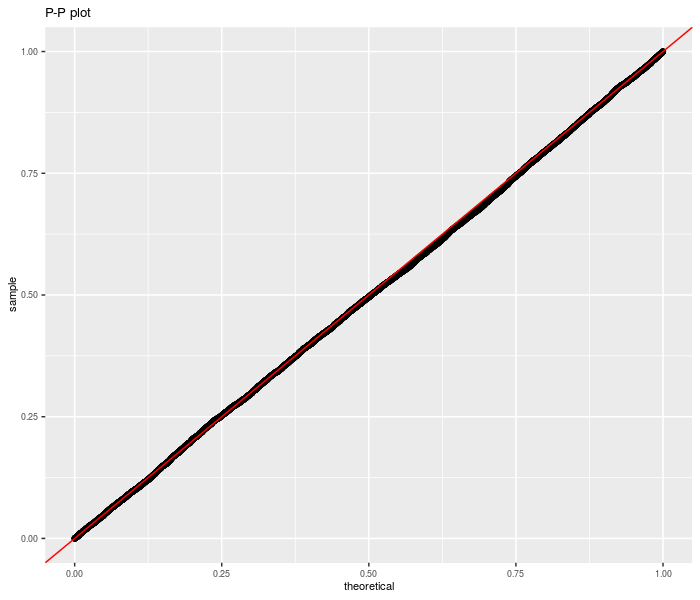# Statistical Significance of the Sharpe Ratio

A collection of tools for analyzing significance of assets, funds, and trading strategies, based on the Sharpe ratio and overfit of the same. Provides density, distribution, quantile and random generation of the Sharpe ratio distribution based on normal returns, as well as the optimal Sharpe ratio over multiple assets. Computes confidence intervals on the Sharpe and provides a test of equality of Sharpe ratios based on the Delta method.

A number of utilities for dealing with Sharpe ratio, the Sharpe ratio of the Markowitz portfolio, and, in general, overfit of trading strategies based on (in-sample) Sharpe statistics.

-- Steven E. Pav, [email protected]gmail.com

## Installation

This package may be installed from CRAN; the latest development version may be installed via drat, or built from github:

# Basic Usage

## Using sr

``````##        SR/sqrt(yr) Std. Error t value Pr(>t)
## Sharpe        1.30       0.46     2.8 0.0023 **
## ---
## Signif. codes:  0 '***' 0.001 '**' 0.01 '*' 0.05 '.' 0.1 ' ' 1
``````

And using real data:

``````##      SR/sqrt(yr) Std. Error t value Pr(>t)
## IBM         0.43       0.32     1.3 0.0905 .
## AAPL        1.09       0.32     3.4 0.0004 ***
## XOM         0.43       0.32     1.3 0.0937 .
## ---
## Signif. codes:  0 '***' 0.001 '**' 0.01 '*' 0.05 '.' 0.1 ' ' 1
``````

## Unpaired test for Sharpe Ratio

A single equation on multiple signal-noise ratios with independent samples can be computed using the `sr_unpaired_test` function. This code performs inference via the Upsilon distribution. The `sr_test` also acts as a frontend for this code, for the two sample case.

First we perform this test under the null, using randomly generated data. We are testing the sum of three differences of Sharpes here.Now we repeat for non-zero null value:Now for real data. We take monthly returns of the three Fama French factors plus momentum (the original Fifth Beatle), then divide into January and non-January periods. We regress Momentum against the other three factors, then convert the linear regression to a Sharpe ratio estimate. The two Sharpe ratios are then thrown into an unpaired sample test. We reject the null of equal idiosyncratic momentum in January versus the rest of the year at the 0.05 level. Is this 'the January Effect'? Perhaps.

``````##
## 	unpaired k-sample sr-test
##
## data:  list(sr.jan, sr.rem)
## df = 90, NA = 1000, p-value = 0.002
## alternative hypothesis: true weighted sum of signal-noise ratios is not equal to 0
## 95 percent confidence interval:
##  -2.34 -0.52
## sample estimates:
## equation on Sharpe ratios
##                      -1.4
``````

## Prediction Intervals

Using the Upsilon distribution, we can compute prediction intervals for future realized Sharpe ratio, with coverage frequency over the full experiment. Here is an example on fake data:

``````##  0.95
``````For a more complicated example, consider the 'Sharpe' under the attribution model. Here we use the daily data of the three Fama French factors, then perform an attribution of SMB against the market and HML. We compute the factor model Sharpe for the first nine months of each year, and of the last three months separately. Using the first three quarters, we compute a prediction interval for the fourth quarter, then check coverage:

``````##  0.8
``````It is not clear if non-normality or omitted variable bias (or broken code!) is to blame for the apparent conservatism of the prediction intervals in this case. We can check by simply shuffling the monthly returns data and repeating the experiment:

``````##  0.92
``````

Of course, this could be a 'lucky seed', but one suspects that non-normality is not the issue, rather there is some autocorrelation of (idiosyncratic) returns (or volatility!).

## Inference on the Markowitz Portfolio

The (negative) Markowitz portfolio appears in the inverse of the uncentered second moment matrix of the 'augmented' vector of returns. Via the Central Limit Theorem and the delta method the asymptotic distribution of the Markowitz portfolio can be found. From this, Wald statistics on the individual portfolio weights can be computed. Here I perform this computation on the portfolio consisting of three large cap stocks, and find that the Markowitz weighting of AAPL is significantly non-zero (modulo the selection biases in universe construction). The results are little changed when using a 'robust' covariance estimator.

``````##        IBM AAPL  XOM
## [1,] -0.27    3 0.15
``````
``````##        IBM AAPL  XOM
## [1,] -0.26    3 0.16
``````

# Reference manual

install.packages("SharpeR")

1.2.0 by Steven E. Pav, a year ago

https://github.com/shabbychef/SharpeR

Report a bug at https://github.com/shabbychef/SharpeR/issues

Browse source code at https://github.com/cran/SharpeR

Authors: Steven E. Pav [aut, cre]

Documentation:   PDF Manual

Task views: Empirical Finance